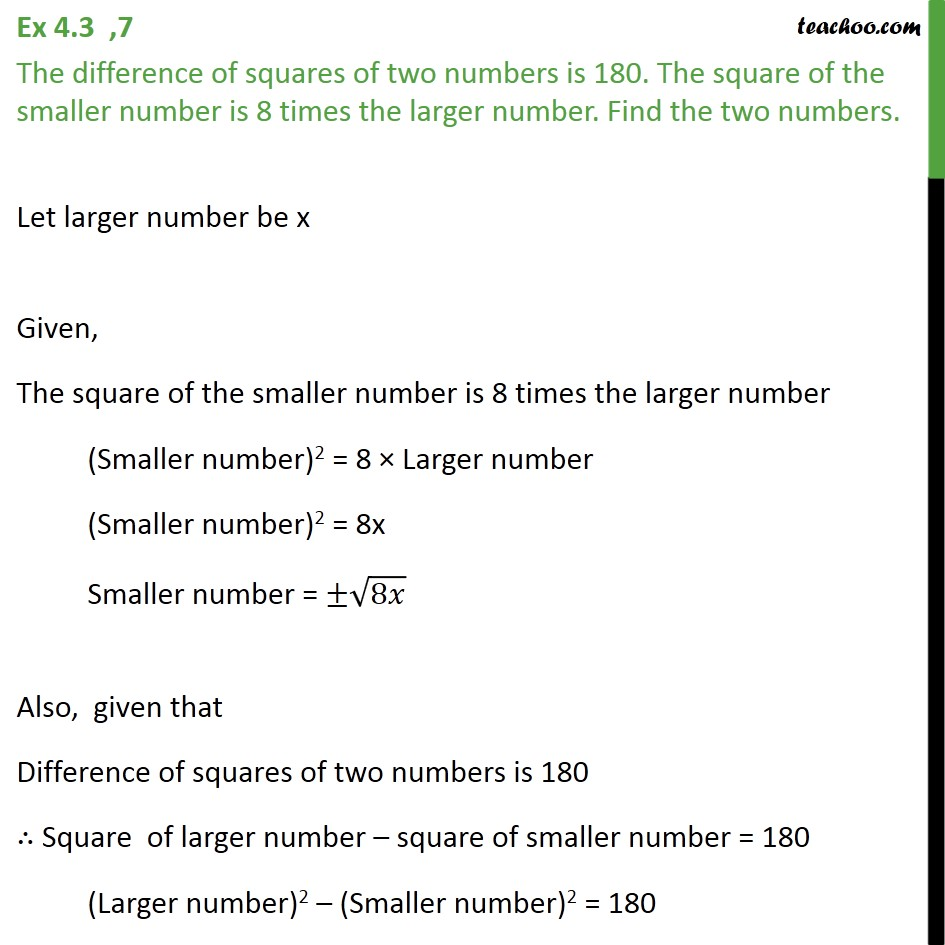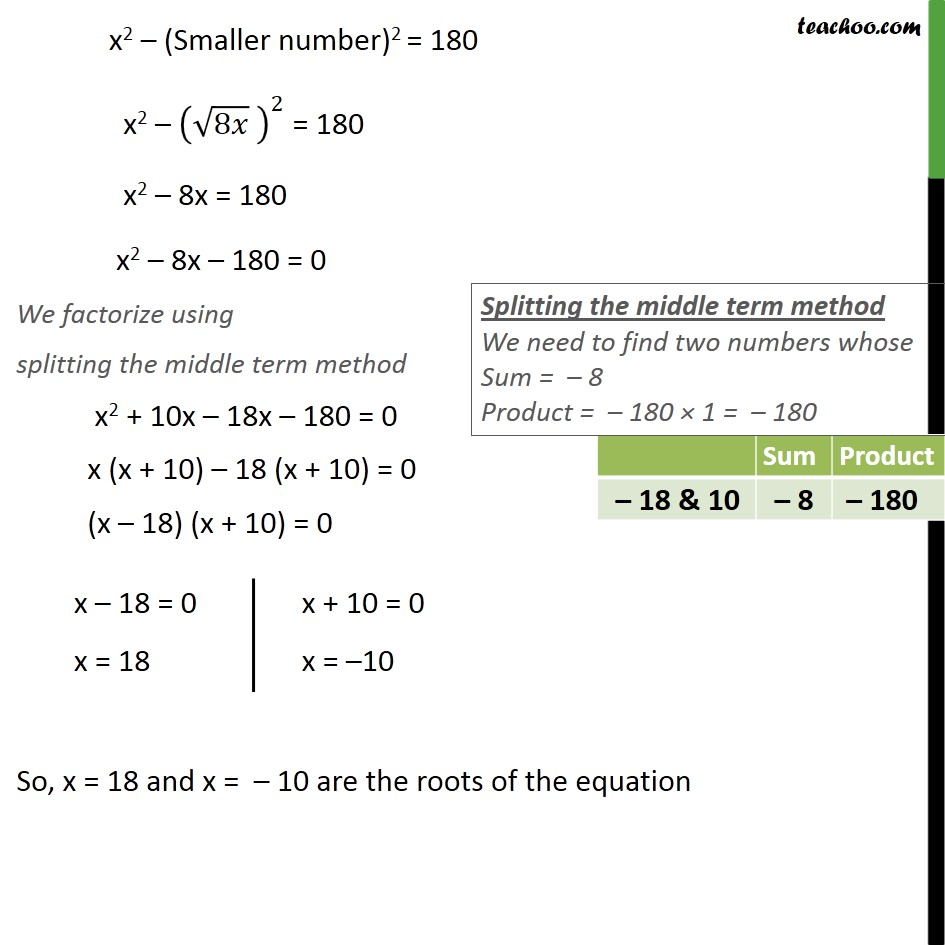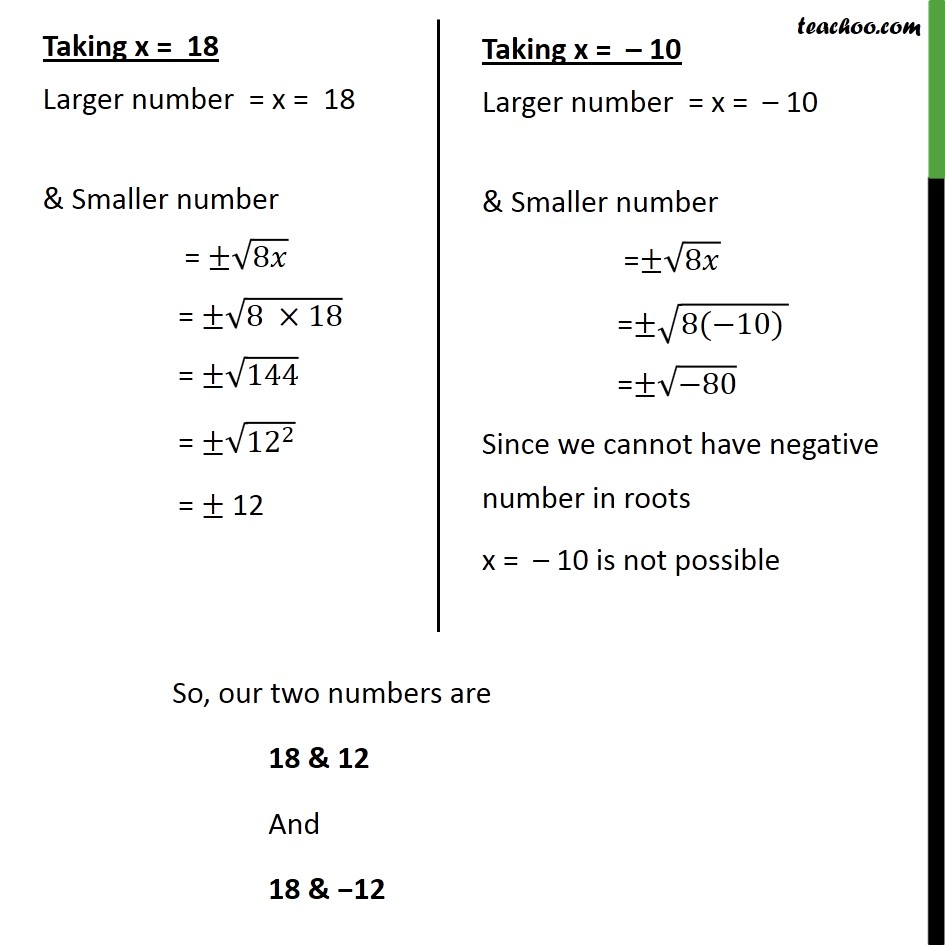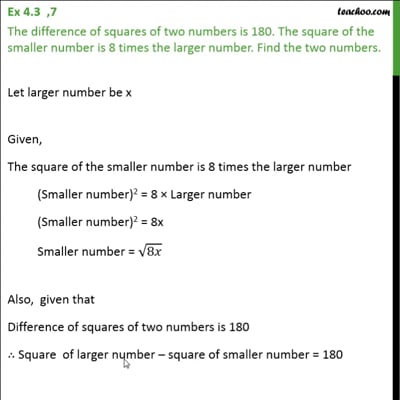Ex 4.3

Chapter 4 Class 10 Quadratic Equations
Serial order wiseThis video is only available for Teachoo black users

Solve all your doubts with Teachoo Black (new monthly pack available now!)

### Transcript

Ex 4.3 ,7 The difference of squares of two numbers is 180. The square of the smaller number is 8 times the larger number. Find the two numbers. Let larger number be x Given, The square of the smaller number is 8 times the larger number (Smaller number)2 = 8 × Larger number (Smaller number)2 = 8x Smaller number = ±√8𝑥 Also, given that Difference of squares of two numbers is 180 ∴ Square of larger number – square of smaller number = 180 (Larger number)2 – (Smaller number)2 = 180 x2 – (Smaller number)2 = 180 x2 – (√8𝑥 )^2 = 180 x2 – 8x = 180 x2 – 8x – 180 = 0 We factorize using splitting the middle term method x2 + 10x – 18x – 180 = 0 x (x + 10) – 18 (x + 10) = 0 (x – 18) (x + 10) = 0 So, x = 18 and x = – 10 are the roots of the equation Taking x = 18 Larger number = x = 18 & Smaller number = ±√8𝑥 = ±√(8 ×18) = ±√144 = ±√(〖12〗^2 ) = ± 12 Taking x = – 10 Larger number = x = – 10 & Smaller number =±√8𝑥 =±√(8(−10) ) =±√(−80) Since we cannot have negative number in roots x = – 10 is not possible So, our two numbers are 18 & 12 And 18 & −12